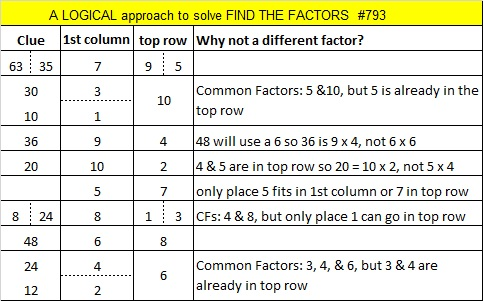# 793 and Level 5

793 is the sum of two squares TWO different ways!

• 28² + 3² = 793
• 27² + 8² = 793

Notice that 793 is 4(198) + 1, and neither 28 and 3 or 27 and 8 have any common prime factors. Could 793 possibly be a prime number?

The answer is no for two reasons:

1. √793 is about 28.2, so we only need to check to see if 793 is divisible by 5, 13, or 17 (all the 4N+1 prime numbers that are less than 28.) However, 793 ÷ 13 = 61, so  793 isn’t prime.
2. Also, any number that can be written as the sum of two squares in more than one way is never a prime number.

Both 13 and 61 give a remainder of one when they are divided by four, and 793 is the hypotenuse of FOUR Pythagorean triples:

• 143-780-793, which is 13 times 11-60-61
• 168-775-793, a primitive calculated from 2(28)(3), 28² – 3², 28² + 3²
• 305-732-793, which is 61 times 5-12-13
• 432-665-793, a primitive calculated from 2(27)(8), 27² – 8², 27² + 8²

793 is also palindrome 191 in BASE 24; note that 1(24²) + 9(24) + 1(1) = 793

Finally, OEIS.org informs us that 793 is 2(397) – 1.Print the puzzles or type the solution on this excel file: 10-factors-788-794

————————————–

• 793 is a composite number.
• Prime factorization: 793 = 13 x 61
• The exponents in the prime factorization are 1 and 1. Adding one to each and multiplying we get (1 + 1)(1 + 1) = 2 x 2 = 4. Therefore 793 has exactly 4 factors.
• Factors of 793: 1, 13, 61, 793
• Factor pairs: 793 = 1 x 793 or 13 x 61
• 793 has no square factors that allow its square root to be simplified. √793 ≈ 28.16025568.This site uses Akismet to reduce spam. Learn how your comment data is processed.